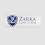# Laws of perfect gas in thermodynamics | Boyle's law | Charle's law | Gay-lussac's law

## A perfect gases (or an ideal gas) may be defined as a state of substance, whose evaporation from in liquid state is complete. It may be noted that if its evaporation is partial, the substance is called vapour. A vapour contains some particles of liquid in suspension. The behaviour of superheated vapours in similar to that of a perfect gas.

The physical properties of a gas are controlled by the following three variables.

1. Pressure exerted by the gas 2. Volume occupied by the gas 3. Temperature of the gas.

The behaviour of a perfect gas, undergoing any change in these three variables, is governed by the following laws.

1. Boyle's laws - Robert Boyle, a British chemist gave the first gas law, now known as Boyles's law. This law describes the relationship between the pressure and volume of a sample of gas confined in a container. Boyle observed that when the pressure on an ideal gas in increased volume decreases. Similarly when pressure is released the volume starts to increase. But Boyle's laws is true only when the temperature of the gas remains constant and no additional gas is added to the container or leaks out of the container. On the basis of these observations the Boyle's law is started as:

"Boyle's law states that the volume and pressure of a sample of gas are inversely proportional to each other at constant temperature".

The statement can be expressed as follows.

α 1 /P    or  PV = k or  V= k 1/P

Where  is the volume P is pressure

2. Charles's Law - Jacques Charles carried out experiments on ideal gas and observed a relationship between the absolute temperature and volume of gases at constant pressure. Volume of the gas increases with an increase in temperature and decrease with a decrease in temperature. The Chale's law  can be started as:

"Charles's  law states that the volume of a sample of gas is directly proportional to the absolute temperature when pressure remains constant".

Charles's law can be expressed as follows.

V α T  OR   V/T =K    OR V=K.T

Where V  is the volume and T is the absolute temperature of the gas.

3. Gay-Lussac's Law -  Pressure of a confined gas increases with increasing temperature. If the temperature of the gas increases enough, the container can explode because of the pressure that builds up inside of it. The relationship between the pressure and temperature of a gas is described by Gay-Lussac's Law "Gay-Lussac's Law states that the pressure of a sample of gas is directly proportional to the absolute temperature when volume remains constant" .

Gay-Lussac's Law can be expressed as follow.

P α T   OR  P/T =K  OR P=K.T

Where P is the pressure and T is the temperature of the gas.

#### 1 comment:

1.I am grateful that I was able to learn something useful from this article. After reading it, I believe you possess excellent expertise. Thank you for sharing that. Keep up the good work. law firm

Thank you for the comment